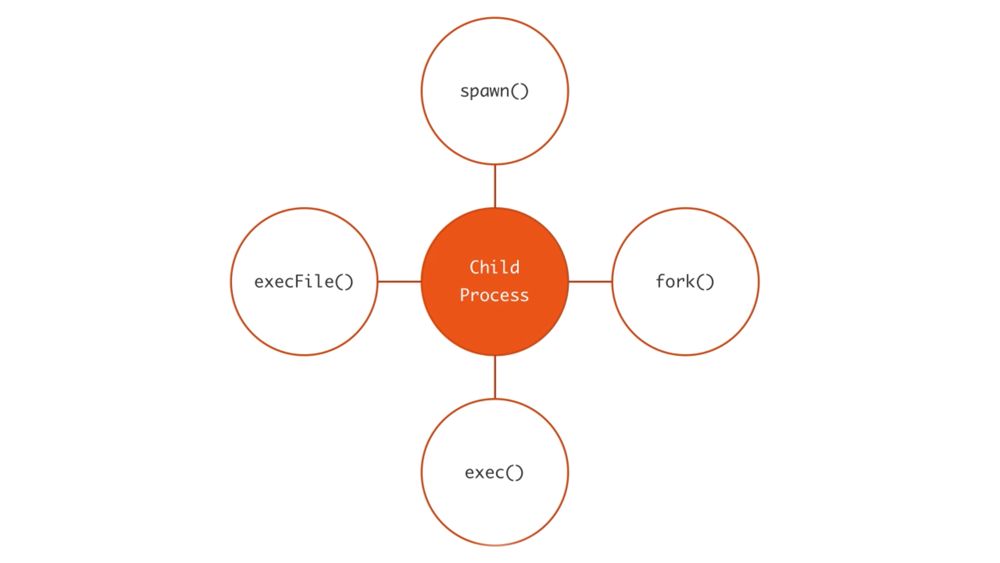https://medium.freecodecamp.org/node-js-child-processes-everything-you-need-to-know-e69498fe970amedium.freecodecamp.org

Node.js 中在单进程中单线程，非阻塞的性能非常好。但是，一个 CPU 一个进程不足以处理程序中日益增加的工作负载。

## 子进程模块

child_process 模块使我们在一个运行良好的子进程内运行一些能够进入操作系统的命令。

## 衍生一个子进程

spawn 函数在一个新的进程里启动一个命令，可以给这个命令传递任何的参数。例如，这里有一段代码衍生一个新进程并且执行 pwd 命令。

``````const {spawn } = require('child_process');
const child = spawn('pwd');
``````

``````child.on('exit', function(code, signal){
console.log('child process exited with' + `code \${code} and signal \${signal}`);
});
``````

• 当父进程手动调用 child.discount 的时候，discount 事件会被触发。
• 如果进程不能被衍生（spawn）或者被 killed，error 事件被触发。
• 当一个子进程的 stdio 关闭的时候，close 事件被触发。
• message 事件是最重要的一个。当子进程使用 process.send() 函数发送信息的时候，message 事件会被触发。这就是父子进程相会交流的方式。我们将会在下面看到一个例子。

``````child.stdout.on('data', (data) => {
console.log(`child stdout: \${data}`)
});
child.stderr.on('data', (data) => {
console.error(`stderror \${data}`);
});
``````

``````const child = spawn('find', ['.', '-type', 'f']);
``````

``````const {spawn} = require('child_process');
const child = spawn('wc');
process.stdin.pipe(child.stdin);
child.stdout.on('data', (data) => {
console.log(`child stdout: \${data}`);
});
``````

``````const {spawn} = require('child_process');
const find = spawn('find', ['.', '-type', 'f']);
const wc = spawn('wc', ['-l']);
find.stdout.pipe(wc.stdin);
wc.stdout.on('data', (data) => {
console.log(`Number of files \${data}`)
});
``````

## shell 语法和 exec 函数

``````const { exec } = require('child_process');

exec('find . -type f | wc -l', (err, stdout, stderr) => {
if (err) {
console.error(`exec error: \${err}`);
return;
}

console.log(`Number of files \${stdout}`);
});
``````

exec 函数将输出放入缓存区，并且将它作为 stdout 传递给回调函数（exec 函数的第二个参数）。stdout 是我们想要打印的命令的输出。

``````const child = spawn('find . -type f | wc -l', {
stdio: 'inherit',
shell: true
});
``````

``````const child = spawn('find . -type f | wc -l', {
stdio: 'inherit',
shell: true,
});
``````

``````const child = spawn('echo \$ANSWER', {
stdio: 'inherit',
shell: true,
});
``````

``````setTimeout(() => {
// keep the event loop busy
}, 20000);
``````

``````const { spawn } = require('child_process');
const child = spawn('node', ['timer.js'], {
detached: true,
stdio: 'ignore'
});
child.unref();
``````

## *Sync 函数

``````const {
spawnSync,
execSync,
execFileSync,
} = require('child_process');
``````

## fork() 函数

fork 函数是spawn 函数的另一种衍生（fork） node 进程的形式。spawn 和 fork 之间最大的不同是当使用 fork 函数时，到子进程的通信通道被建立了，因此我们可以在子进程里通过全局的 process 使用 send 函数，在父子进程之间交换信息。通过 EventEmitter 模块接口实现的。下面是例子：

parent.js

``````const {fork} = require('child_process');
const forked = fork('child.js');
forked.on('message', (msg) => {
console.log('messsgae from child', msg);
});

forked.send({hello: 'world'});
``````

child.js

``````process.on('message', (msg) => {
console.log('message from parent:', msg);
});
let conter = 0;
setInterval(() => {
process.send({counter: counter++});
}, 1000);
``````

``````const http = require('http');
const longComputation = () => {
let sum = 0;
for (let i = 0; i<1e9; i++) {
sum += i;
}
return sum;
};

const server = http.createServer();
server.on('request', (req, res) => {
if (req.url === '/compute') {
const sum = longComputation();
return res.end(`Sum is \${sum}`);
} else {
res.end('Ok');
}
});
server.listen(3000);
``````

``````const longComputation = () => {
let sum = 0;
for (let i = 0; i< 1e9;i++) {
sum += i;
}
return sum;
};

process.on('message', (msg) => {
const sum = longComputation();
process.send(sum);
});
``````

``````const http = require('http');
const { fork } = require('child_process');
const server = http.createServer();

server.on('request', (req, res) => {
if(req.url === '/compute') {
const compute = fork('compute.js');
compute.send('start');
compute.on('message', sum => {
res.end(`Sum is \${sum}`)
})
} else {
res.end('OK');
}
});
server.listen(3000);
``````

Node 的 cluster 模块，基于子进程的理念并且在多个子进程之间负载，可以在任意的操作系统上面创建。